# From Relational Calculus to Relational Algebra Tuple relational

• Slides: 24
Download presentationFrom Relational Calculus to Relational Algebra Tuple relational calculus, domain relational calculus, and relational algebra 9/17/2020 1 CS 319 Theory of Databases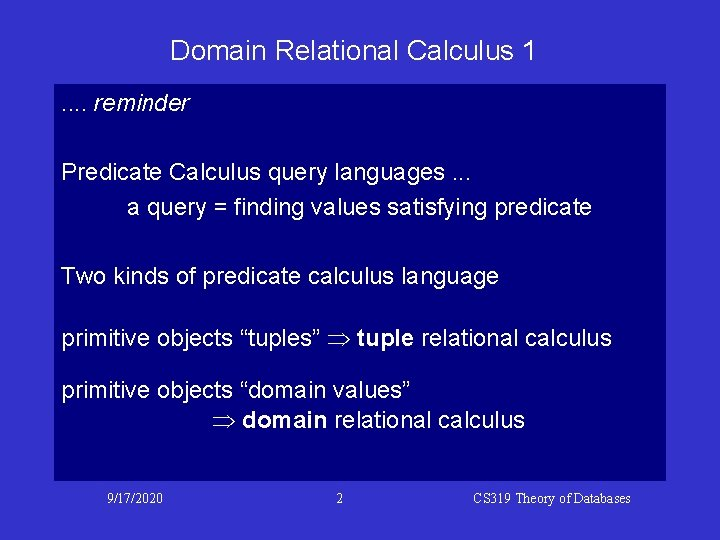Domain Relational Calculus 1. . reminder Predicate Calculus query languages. . . a query = finding values satisfying predicate Two kinds of predicate calculus language primitive objects “tuples” tuple relational calculus primitive objects “domain values” domain relational calculus 9/17/2020 2 CS 319 Theory of Databases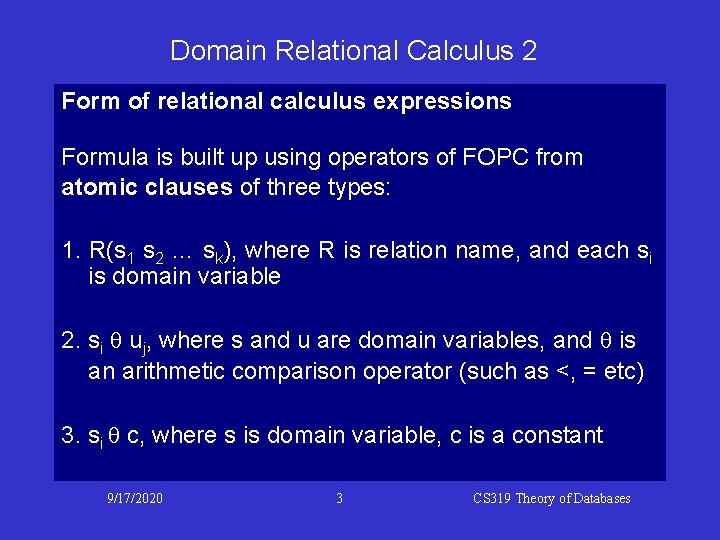Domain Relational Calculus 2 Form of relational calculus expressions Formula is built up using operators of FOPC from atomic clauses of three types: 1. R(s 1 s 2 … sk), where R is relation name, and each si is domain variable 2. si q uj, where s and u are domain variables, and q is an arithmetic comparison operator (such as <, = etc) 3. si q c, where s is domain variable, c is a constant 9/17/2020 3 CS 319 Theory of Databases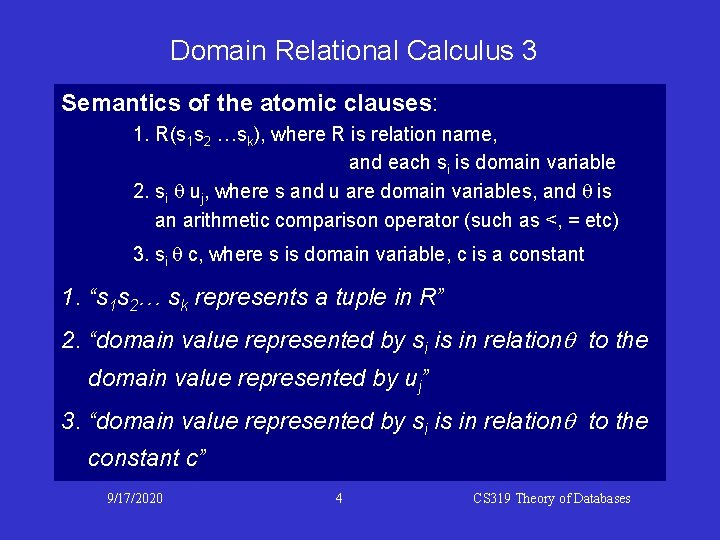Domain Relational Calculus 3 Semantics of the atomic clauses: 1. R(s 1 s 2 …sk), where R is relation name, and each si is domain variable 2. si q uj, where s and u are domain variables, and q is an arithmetic comparison operator (such as <, = etc) 3. si q c, where s is domain variable, c is a constant 1. “s 1 s 2… sk represents a tuple in R” 2. “domain value represented by si is in relationq to the domain value represented by uj” 3. “domain value represented by si is in relationq to the constant c” 9/17/2020 4 CS 319 Theory of DatabasesFrom tuple to domain relational calculus 1 The definition of safety is expressed in terms of constraints on component values of tuples. . . so generalises directly to domain relational calculus Theorem 2: For every safe tuple relational calculus expression there is an equivalent safe domain relational calculus expression. Omit formal proof: essentially a syntactic transformation involving substitution for tuple variables 9/17/2020 5 CS 319 Theory of Databases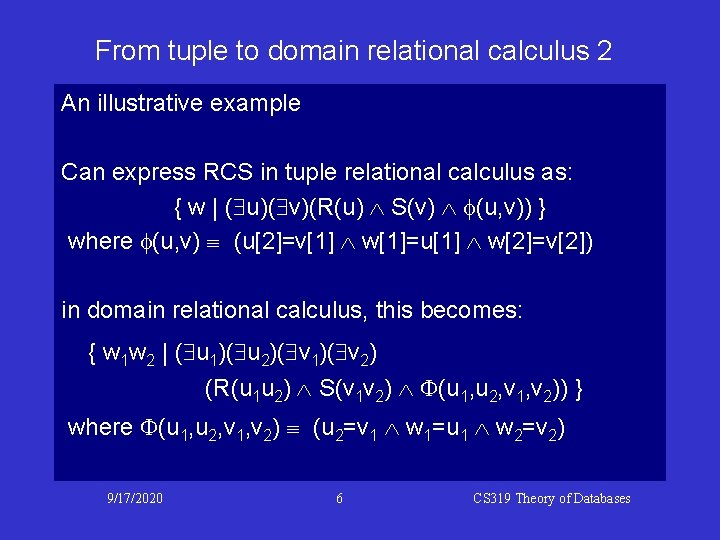From tuple to domain relational calculus 2 An illustrative example Can express RCS in tuple relational calculus as: { w | ( u)( v)(R(u) S(v) f(u, v)) } where f(u, v) (u=v w=u w=v) in domain relational calculus, this becomes: { w 1 w 2 | ( u 1)( u 2)( v 1)( v 2) (R(u 1 u 2) S(v 1 v 2) F(u 1, u 2, v 1, v 2)) } where F(u 1, u 2, v 1, v 2) (u 2=v 1 w 1=u 1 w 2=v 2) 9/17/2020 6 CS 319 Theory of DatabasesFrom domain relational calculus to algebra 1 Theorem 3: For every safe expression in domain relational calculus, there is an equivalent relational algebra expression. Proof (sketch only) Use induction on number of operators in y to construct an algebraic expression for { t 1 t 2. . . tn | y(t 1, t 2, . . . , tn) } To simplify the induction, begin with two lemmas: • there is a relational algebra exp to represent dom(y) • don’t need to consider and as independent cases 9/17/2020 7 CS 319 Theory of Databases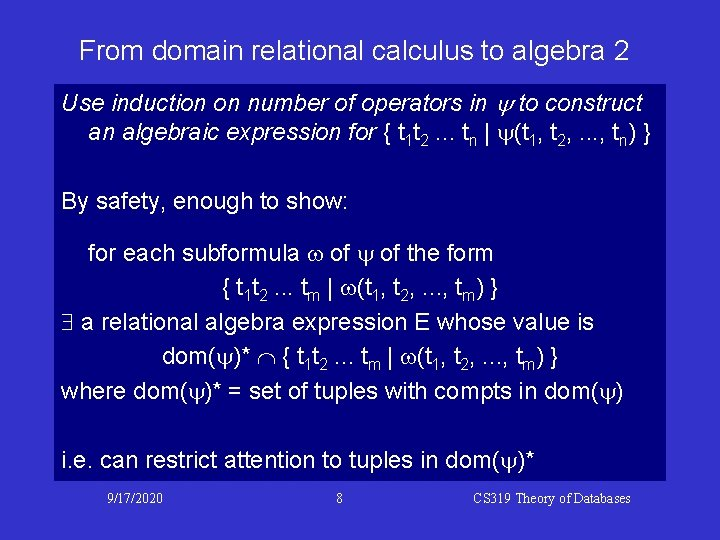From domain relational calculus to algebra 2 Use induction on number of operators in y to construct an algebraic expression for { t 1 t 2. . . tn | y(t 1, t 2, . . . , tn) } By safety, enough to show: for each subformula w of y of the form { t 1 t 2. . . tm | w(t 1, t 2, . . . , tm) } a relational algebra expression E whose value is dom(y)* Ç { t 1 t 2. . . tm | w(t 1, t 2, . . . , tm) } where dom(y)* = set of tuples with compts in dom(y) i. e. can restrict attention to tuples in dom(y)* 9/17/2020 8 CS 319 Theory of DatabasesFrom domain relational calculus to algebra 3 Lemma A: If y is any formula in domain relational calculus, there is a relational algebra expression to represent the unary relation dom(y) Note: unary relation set of 1 -tuples set Proof: Suppose R has arity k. Let D(R) P 1(R) P 2(R) . . . Pk(R). dom(y) is the union of all D(R)'s over relations R referred to in y together with the set of all constants {a 1, a 2, . . . , an} referred to in y. Thus can take D as an algebraic expression: D= 9/17/2020 R referred to in y D(R) {a 1, a 2, . . . , an} 9 CS 319 Theory of Databases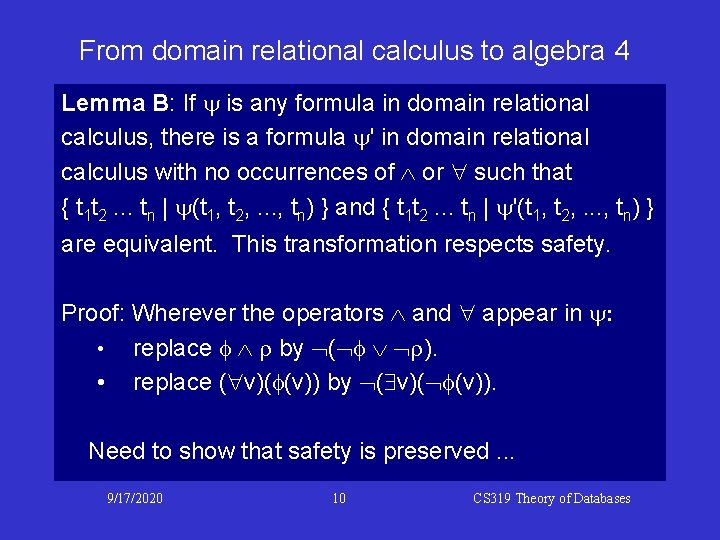From domain relational calculus to algebra 4 Lemma B: If y is any formula in domain relational calculus, there is a formula y' in domain relational calculus with no occurrences of or such that { t 1 t 2. . . tn | y(t 1, t 2, . . . , tn) } and { t 1 t 2. . . tn | y'(t 1, t 2, . . . , tn) } are equivalent. This transformation respects safety. Proof: Wherever the operators and appear in y: • replace f r by ( f r). • replace ( v)(f(v)) by ( v)( f(v)). Need to show that safety is preserved. . . 9/17/2020 10 CS 319 Theory of Databases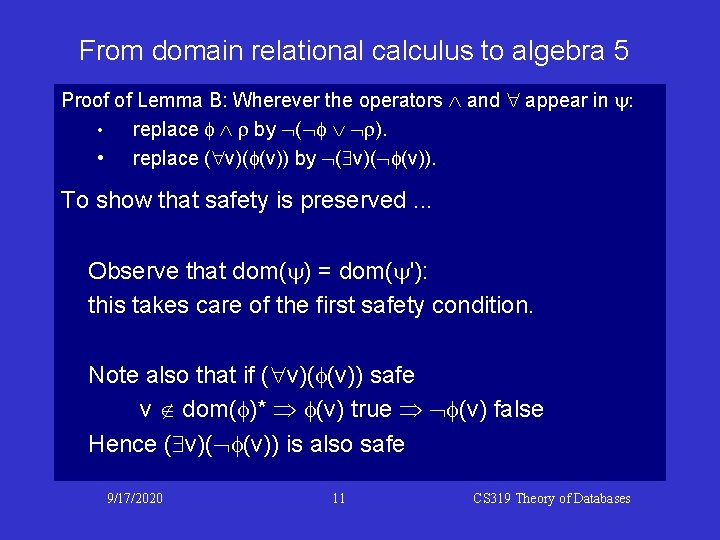From domain relational calculus to algebra 5 Proof of Lemma B: Wherever the operators and appear in y: • replace f r by ( f r). • replace ( v)(f(v)) by ( v)( f(v)). To show that safety is preserved. . . Observe that dom(y) = dom(y'): this takes care of the first safety condition. Note also that if ( v)(f(v)) safe v Ï dom(f)* f(v) true f(v) false Hence ( v)( f(v)) is also safe 9/17/2020 11 CS 319 Theory of Databases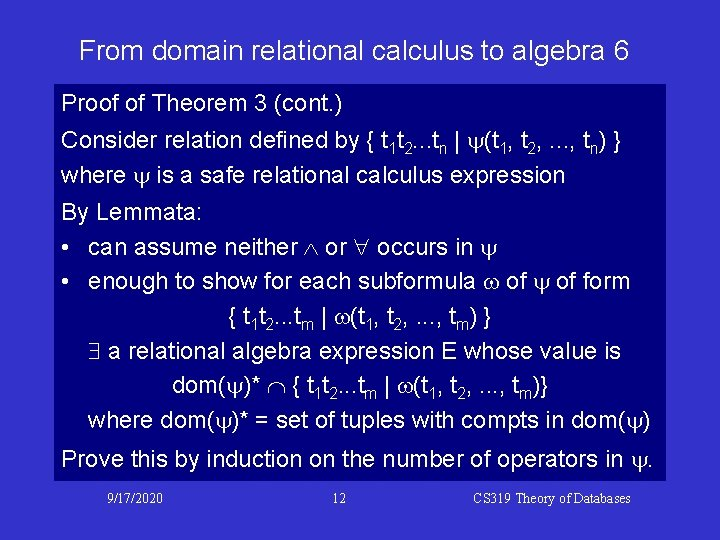From domain relational calculus to algebra 6 Proof of Theorem 3 (cont. ) Consider relation defined by { t 1 t 2. . . tn | y(t 1, t 2, . . . , tn) } where y is a safe relational calculus expression By Lemmata: • can assume neither occurs in y • enough to show for each subformula w of y of form { t 1 t 2. . . tm | w(t 1, t 2, . . . , tm) } a relational algebra expression E whose value is dom(y)* Ç { t 1 t 2. . . tm | w(t 1, t 2, . . . , tm)} where dom(y)* = set of tuples with compts in dom(y) Prove this by induction on the number of operators in y. 9/17/2020 12 CS 319 Theory of DatabasesFrom domain relational calculus to algebra 7 I. e. prove for all subformulae w of y in particular for y itself by induction on number of operators N in w. N=0: consider the relation defined by dom(y)* Ç { t 1 t 2. . . tm | w(t 1, t 2, . . . , tm) } where w is an atomic formula. Let D be relational algebra expression for dom(y). Two cases: 1. w(ti, tj) = ti q tj or w(ti) = ti q c where q is an arithmetic comparison operator 2. w(t 1, t 2, . . . , tm) = R(ti(1)ti(2). . . ti(k)) 9/17/2020 13 CS 319 Theory of Databases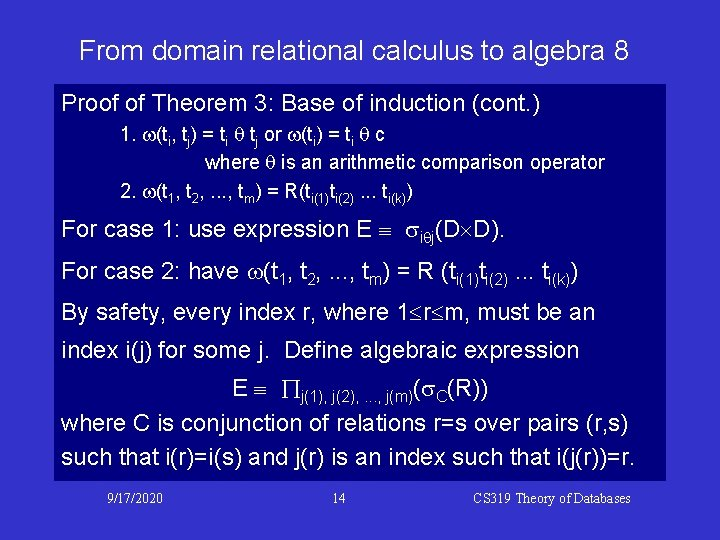From domain relational calculus to algebra 8 Proof of Theorem 3: Base of induction (cont. ) 1. w(ti, tj) = ti q tj or w(ti) = ti q c where q is an arithmetic comparison operator 2. w(t 1, t 2, . . . , tm) = R(ti(1)ti(2). . . ti(k)) For case 1: use expression E siqj(D´D). For case 2: have w(t 1, t 2, . . . , tm) = R (ti(1)ti(2). . . ti(k)) By safety, every index r, where 1 r m, must be an index i(j) for some j. Define algebraic expression E Õj(1), j(2), . . . , j(m)(s. C(R)) where C is conjunction of relations r=s over pairs (r, s) such that i(r)=i(s) and j(r) is an index such that i(j(r))=r. 9/17/2020 14 CS 319 Theory of Databases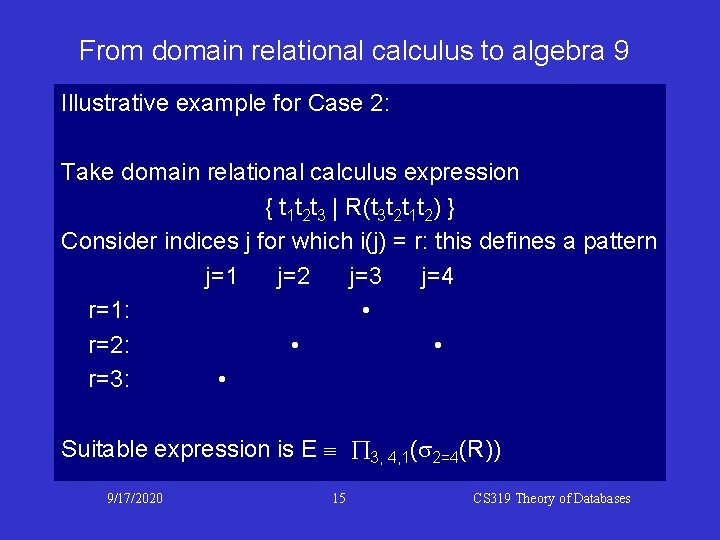From domain relational calculus to algebra 9 Illustrative example for Case 2: Take domain relational calculus expression { t 1 t 2 t 3 | R(t 3 t 2 t 1 t 2) } Consider indices j for which i(j) = r: this defines a pattern j=1 j=2 j=3 j=4 r=1: • r=2: • • r=3: • Suitable expression is E Õ 3, 4, 1(s 2=4(R)) 9/17/2020 15 CS 319 Theory of Databases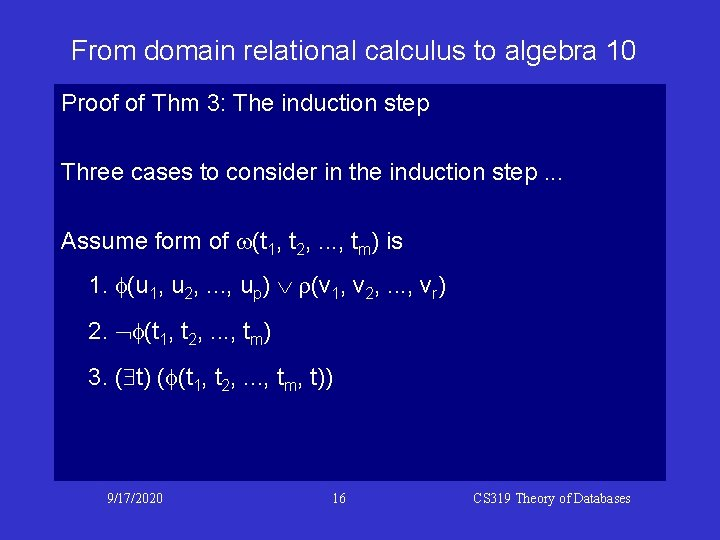From domain relational calculus to algebra 10 Proof of Thm 3: The induction step Three cases to consider in the induction step. . . Assume form of w(t 1, t 2, . . . , tm) is 1. f(u 1, u 2, . . . , up) r(v 1, v 2, . . . , vr) 2. f(t 1, t 2, . . . , tm) 3. ( t) (f(t 1, t 2, . . . , tm, t)) 9/17/2020 16 CS 319 Theory of Databases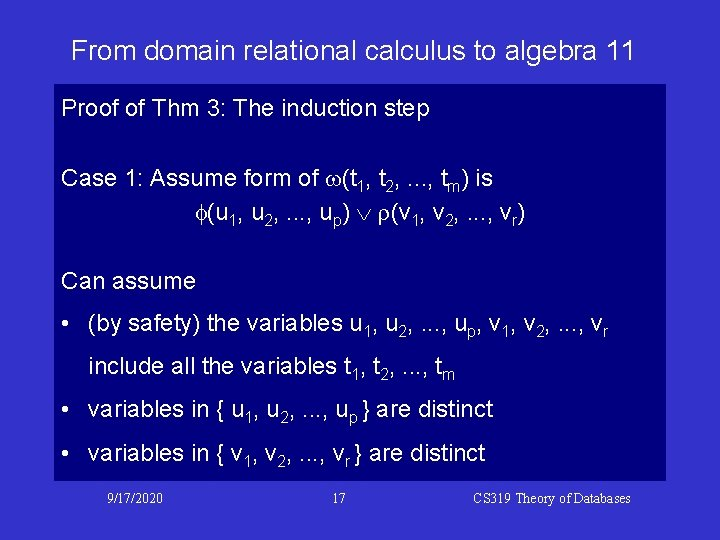From domain relational calculus to algebra 11 Proof of Thm 3: The induction step Case 1: Assume form of w(t 1, t 2, . . . , tm) is f(u 1, u 2, . . . , up) r(v 1, v 2, . . . , vr) Can assume • (by safety) the variables u 1, u 2, . . . , up, v 1, v 2, . . . , vr include all the variables t 1, t 2, . . . , tm • variables in { u 1, u 2, . . . , up } are distinct • variables in { v 1, v 2, . . . , vr } are distinct 9/17/2020 17 CS 319 Theory of Databases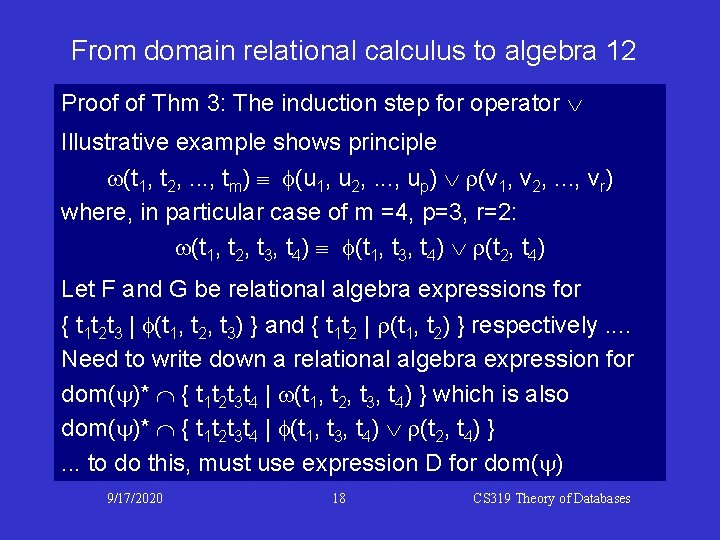From domain relational calculus to algebra 12 Proof of Thm 3: The induction step for operator Illustrative example shows principle w(t 1, t 2, . . . , tm) f(u 1, u 2, . . . , up) r(v 1, v 2, . . . , vr) where, in particular case of m =4, p=3, r=2: w(t 1, t 2, t 3, t 4) f(t 1, t 3, t 4) r(t 2, t 4) Let F and G be relational algebra expressions for { t 1 t 2 t 3 | f(t 1, t 2, t 3) } and { t 1 t 2 | r(t 1, t 2) } respectively. . Need to write down a relational algebra expression for dom(y)* Ç { t 1 t 2 t 3 t 4 | w(t 1, t 2, t 3, t 4) } which is also dom(y)* Ç { t 1 t 2 t 3 t 4 | f(t 1, t 3, t 4) r(t 2, t 4) }. . . to do this, must use expression D for dom(y) 9/17/2020 18 CS 319 Theory of DatabasesFrom domain relational calculus to algebra 13 Proof of Thm 3: The induction step for operator (cont. ) Need a relational algebra expression for dom(y)* Ç { t 1 t 2 t 3 t 4 | f(t 1, t 3, t 4) r(t 2, t 4) } Set of tuples t 1 t 2 t 3 t 4 satisfying f(t 1, t 3, t 4) is constrained so that t 1 t 3 t 4 is a tuple in the relation defined by algebraic expression F. If D is the algebraic expression for dom(y) then F´D defines tuples t 1 t 3 t 4 t 2 satisfying f(t 1, t 3, t 4) within dom(y)*. Hence Õ 1, 4, 2, 3(F´D) defines tuples t 1 t 2 t 3 t 4 satisfying f(t 1, t 3, t 4) within dom(y)*. 9/17/2020 19 CS 319 Theory of DatabasesFrom domain relational calculus to algebra 14 Proof of Thm 3: The induction step for operator (cont. ) Need a relational algebra expression for dom(y)* Ç { t 1 t 2 t 3 t 4 | f(t 1, t 3, t 4) r(t 2, t 4) } Õ 1, 4, 2, 3(F´D) defines tuples t 1 t 2 t 3 t 4 satisfying f(t 1, t 3, t 4) in dom(y)*. Similarly G ´ D defines tuples t 2 t 4 t 1 t 3 satisfying r(t 2, t 4) within dom(y)* and Õ 3, 1, 4, 2(G ´ D) defines tuples t 1 t 2 t 3 t 4 satisfying r(t 2, t 4) within dom(y)*. Hence can take E Õ 1, 4, 2, 3(F´D) Õ 3, 1, 4, 2(G´D´D) 9/17/2020 20 CS 319 Theory of DatabasesFrom domain relational calculus to algebra 15 Case 2: w(t 1, t 2, . . . , tm) is f(t 1, t 2, . . . , tm) If F is an algebraic expression for dom(y)* Ç { t 1 t 2. . . tm | f(t 1, t 2, . . . , tm) } and D is an algebraic expression for dom(y) then D ´. . . ´ D F m times represents the relation dom(y)* { t 1 t 2. . . tm | f(t 1, t 2, . . . , tm) } = dom(y)* (dom(y)* {t 1 t 2. . . tm | f(t 1, t 2, . . . , tm) }) = dom(y)* Ç { t 1 t 2. . . tm | f(t 1, t 2, . . . , tm) } 9/17/2020 21 CS 319 Theory of Databases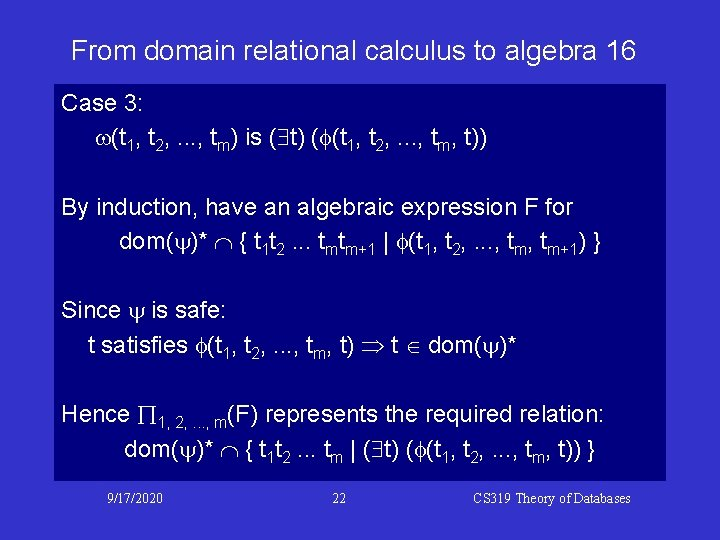From domain relational calculus to algebra 16 Case 3: w(t 1, t 2, . . . , tm) is ( t) (f(t 1, t 2, . . . , tm, t)) By induction, have an algebraic expression F for dom(y)* Ç { t 1 t 2. . . tmtm+1 | f(t 1, t 2, . . . , tm+1) } Since y is safe: t satisfies f(t 1, t 2, . . . , tm, t) t Î dom(y)* Hence Õ 1, 2, . . . , m(F) represents the required relation: dom(y)* Ç { t 1 t 2. . . tm | ( t) (f(t 1, t 2, . . . , tm, t)) } 9/17/2020 22 CS 319 Theory of DatabasesFrom domain relational calculus to algebra 17 Have proved the equivalence of relational algebra and domain / tuple relational calculus. . . Theorems 1, 2 and 3 together prove relational algebra domain relational calculus tuple relational calculus all have the same expressive power. Thus A query language is complete if and only if it has the expressive power of one of these formalisms. 9/17/2020 23 CS 319 Theory of DatabasesTo follow … Mathematical foundations and features of SQL 9/17/2020 24 CS 319 Theory of Databases